# Using Multidimensional Linear Interpolation to find Economic Capital Rates

In banking, Economic Capital (EC), is an internal measure of capital required to absorb unexpected losses while remaining solvent at a targeted solvency level. It provides a common basis for comparing risk-adjusted profitability and relative economic value of lines of business and asset classes with varying degrees and sources of risk.  EC has various applications that include performance measurement, risk-adjusted pricing, capital allocation, capital adequacy and risk concentration management. EC can be allocated at either a loan, facility or line of business level.

Economic Capital is statistically/quantitatively determined and designed to be sensitive to changes in loan characteristics (risk factors) as a result of both systematic and idiosyncratic factors. Very often EC is calculated through the use of Monte Carlo Simulation – An analytical technique that involves performing a large number of random iterations, called simulations, to generate a statistical distribution of possible outcomes.  In finance, Monte Carlo simulations are used to value and analyze complex instruments, portfolios and investments by simulating the various sources of uncertainty affecting their value.

As it relates to EC calculations for banks, the Monte Carlo involves running a large number of simulations of the banking book taking into account each loan’s Probability of Default (PD), Loss Given Default (LGD), remaining term of the loan (Maturity), correlation between the various borrowers in the portfolio, industry, country, etc . .  The result of these simulations is a loss distribution of the portfolio. Economic capital is then estimated as the difference between some given percentile of a loss distribution and the expected loss.

To provide a more tractable method for calculating EC, practitioners typically bucket (discretize) the various simulation inputs, PD, LGD, Correlation, and Maturity as a means to create a segmentation scheme.  Figure 1 illustrates the bucketing for a stylized bank (Kyler Bank).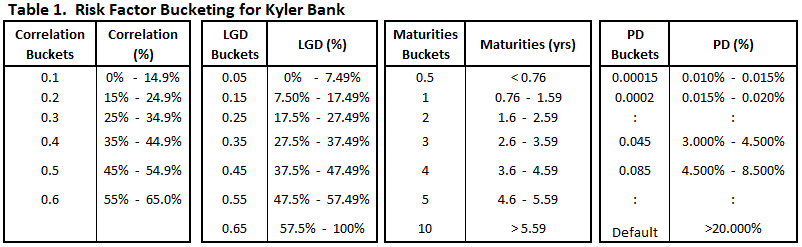This approach produces a single grid (table) containing capital rates for all segments (possible combinations of the aforementioned primary credit risk characteristics).  The grid can then be used to assign capital rates to a facility, based on those characteristics. A typical capital rate grid as in Table 2 below, can sometimes consist of hundreds of thousands of capital rates.Although the grid consists of a large number of segments, not all of the possible combinations of inputs are available in the grid.  This is a consequence of the bucketing of the inputs. Therefore, in order to obtain capital rates for points laying within the bucketed limits a multidimensional interpolation is necessary.  Only multidimensional linear interpolation is considered in this article for several reasons: 1) it’s simplicity,  2) speed of the computation and 2) it is useful for approximating nonlinear solutions.

One dimensional linear interpolation is easy enough to make intuitive sense, but it starts to get murky in higher dimensions.

Interpolation is the process of finding a value between existing discrete points on a line or higher dimensional space. For example, given an array (or table) of values for a function of one or more variables, we often want to find a value between points in the table or values corresponding to combinations of the variables which do not exist in the table.

If the given function is not linear, then the interpolated value will be an approximation. If the array has more than two dimensions, the value sought will be at a point within the interior of the corresponding polytope.  This article is not a mathematical treatise and is only meant to provider readers an example of how they can use linear interpolation to achieve quick acceptable results .

## Linear Interpolation

Below we begin with a simple 1-dimensional linear interpolation and extend it to higher dimensions in subsequent examples. The simplest case of interpolation is in the case of a single dimension.  We can think of 1 dimensional linear interpolation is simply a weighted average of 2 neighboring points.Let’s look at an example, Table 2 contains the EC Rate as a function of probability of default (PD), with all other risk factors held constant:Given the table above we can estimate the EC Rate at 6.5% PD as a weighted-average of the neighboring points or the normalized line segments between the points A and B. Given the points A(x0,y0) and B A(x1,y1) on a line segment in Figure 1, we can find the point C(x,y).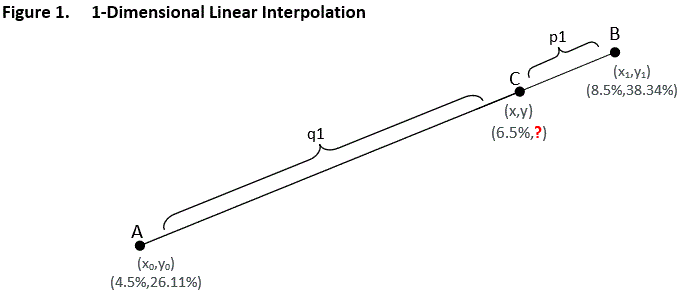The code below demonstrates how we can use simple 1 dimensional interpolation to estimate the EC rate at 6.5% PD.

```
x&nbsp;&nbsp;&lt;- 0.065
x1 &lt;- 0.045
x2 &lt;- 0.085

#values
N1 &lt;- y1 = 0.2611
N2 &lt;- y2 = 0.3834

#weights
q1 &lt;- ( x - x1)/( x2 - x1)&nbsp; &nbsp;&nbsp;&nbsp;&nbsp;&nbsp;&nbsp;&nbsp;&nbsp;&nbsp;&nbsp;&nbsp;&nbsp;&nbsp;&nbsp;‘weight 1
p1 &lt;- 1 - q1&nbsp;&nbsp;&nbsp;&nbsp;&nbsp;&nbsp;&nbsp;&nbsp;&nbsp;&nbsp;&nbsp;&nbsp;&nbsp;&nbsp;&nbsp;&nbsp;&nbsp;&nbsp;&nbsp;&nbsp;&nbsp;&nbsp;&nbsp;&nbsp;&nbsp;&nbsp;&nbsp;&nbsp;&nbsp;&nbsp;‘weight 2

V1d &lt;- p1* N1 + q1* N2
print (V1d)

--------------

&gt; 0.32225
```

## Bi-linear Interpolation

Bi-linear interpolation is an extension of linear interpolation applied to a two dimensional rectangular grid.  A bi-linear interpolation is essentially a linear interpolation of 2 values that are already linearly interpolated values. The rectangular grid is divided into 4 sub-rectangles by the point in question (E). Once the rectangle ABCD is partitioned into four sub-rectangles, the partitions then are normalized by the area of the larger rectangle, ABCD. The weight of each sub-rectangle is given by the area of the opposite sub-rectangle, as a fraction of the whole rectangle.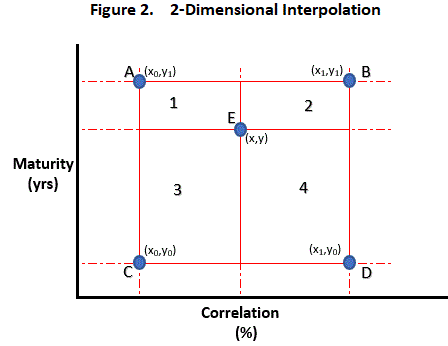Algebraically, the bi-linear interpolation is given by:Let us consider an example where we have a table containing EC rates by Term-to-Maturity and Correlation.  (Note that in this example the Probability of Default (PD) and Loss Given Default (LGD) are assumed to be fixed.)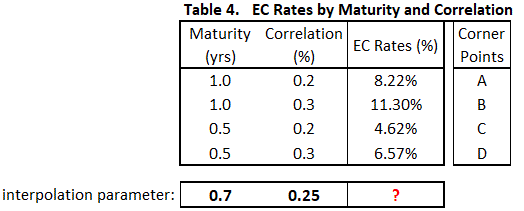If we are given a loan with Maturity = 0.7 years and Correlation = 25%, we can calculate the corresponding EC rate by using bi-linear interpolation.

Figure 3 demonstrates how we can visualize this problem.The following algorithm in R demonstrates how we can calculate the EC rate for the loan in question.

```#interpolation parameters
x&nbsp; &lt;- 0.25     #correlation
y  &lt;- 0.7      #maturity

#correlation
x1 &lt;- 0.2
x2 &lt;- 0.3

#maturity
y1 &lt;- 0.5
y2 &lt;- 1.0

#capital rates
A &lt;- 0.0822
D &lt;- 0.0657
C &lt;- 0.0462
B &lt;- 0.1130&nbsp;

#weights
q1 &lt;- (x - x1)/( x2 - x1)
q2 &lt;- (y - y1)/( y2 - y1)
p1 &lt;- 1 - q1
p2 &lt;- 1 - q2&nbsp;&nbsp;&nbsp;&nbsp;&nbsp;&nbsp;&nbsp;&nbsp;&nbsp;&nbsp;&nbsp;&nbsp;&nbsp;&nbsp;&nbsp;&nbsp;&nbsp;&nbsp;&nbsp;&nbsp;&nbsp;&nbsp;&nbsp;&nbsp;&nbsp;&nbsp;&nbsp;&nbsp;&nbsp;&nbsp;&nbsp;

Nab &lt;- p1*A + q1*B
Ncd &lt;- p1*C + q1*D

V2d &lt;- p2*Nab + q2*Ncd
print(V2d)

-------------

&gt; 0.07426
```

## Tri-linear Interpolation

Tri-linear interpolation is a further extension of linear and bi-linear interpolation applied to a three dimensional rectangular prism. A tri-linear interpolation is a linear interpolation between 2 already bi-linear interpolated values. Tri-linear interpolation is a weighted average of 8 neighboring points.

The prism is divided into sub-rectangular prisms by the planes associated with the point in question (I). Once the prism ABCDEFGH is partitioned into 8 sub-rectangular prisms, the volumes of the partitions then are normalized by the area of the volume of the prism ABCDEFGH. The weight of each neighboring point is given by the area of the opposite sub-rectangular prism, as a fraction of the whole rectangular prism.

Geometrically, the tri-linear interpolation is illustrated by the cube in the Figure 4 :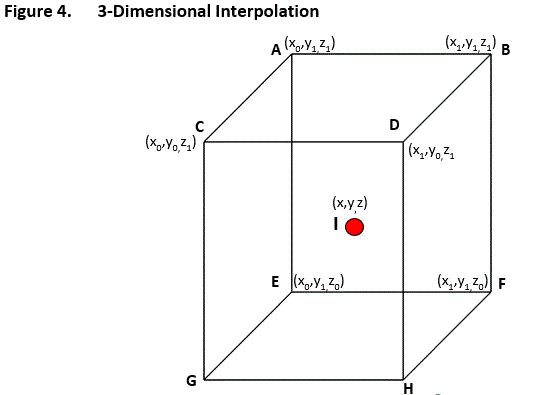Algebraically, The tri-linear interpolation is given by: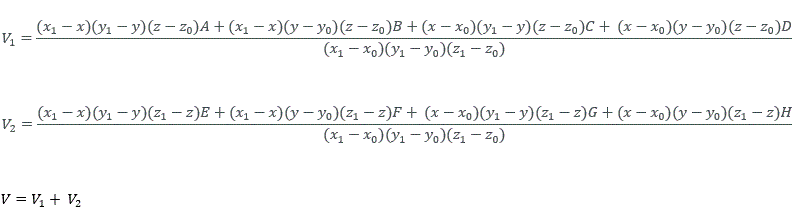To better understand tri-linear interpolation let’s put it in the context of assigning EC rates using a table (grid) of existing EC rates.  As mentioned above, a typical grid of EC rates can contain hundreds of thousands of rates.  For ease of illustration in the following example, we treat PDs as a fixed variable (corresponding to specific FDGs) and therefore only interpolate across the remaining three continuous variables: Correlation, LGD, and Term-to-Maturity.  In our example, we are given a loan with the following characteristics: Maturity = 0.7, Correlation = 25% and LGD = 20%; and want to find the interpolated EC rate.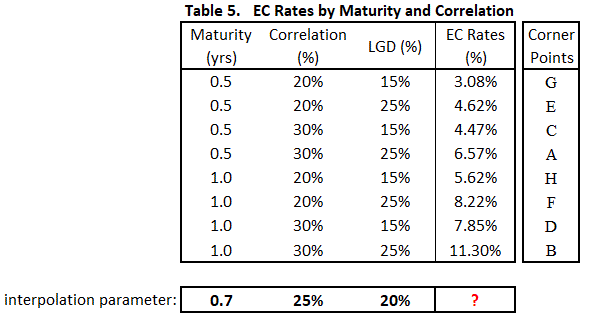The corner points (A through H) in Table 5 correspond to the EC rates boundaries (A through H) displayed in Figure 5a and 5b. It is within these boundaries that the interpolated EC rate for the facility will fall. These EC rate boundaries are then used in a multidimensional linear interpolation routine to produce the desired result.

In this example, we will not consider the variability around the PD characteristic, i.e. we are considering 3 of the 4 continuous variables.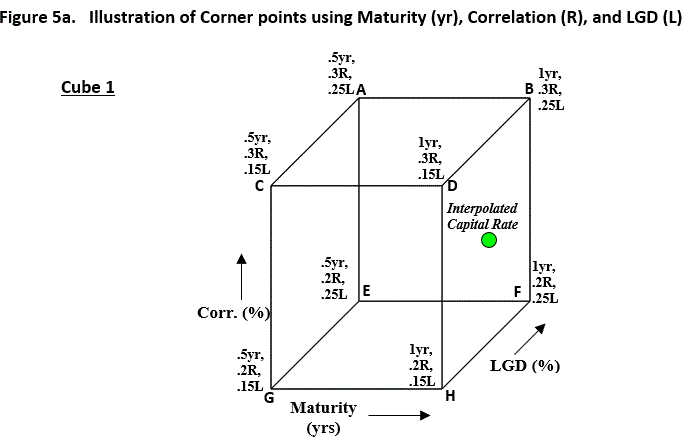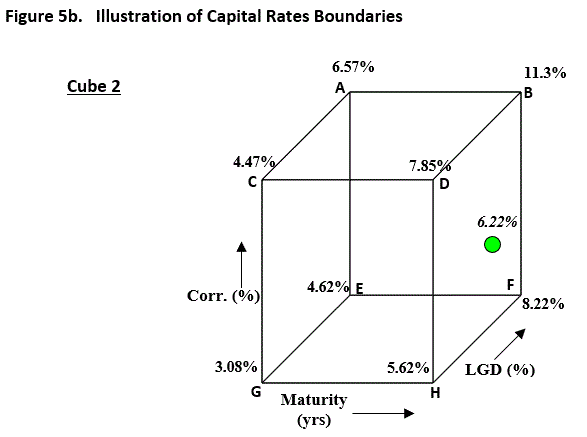```#interpolation parameters
x&nbsp; &lt;- 0.25      #correlation
y&nbsp; &lt;- 0.7       #maturity
z &lt;-&nbsp; 0.20      #lgd

#correlation
x1 &lt;- 0.20
x2 &lt;- 0.30

#maturity
y1 &lt;- 0.5
y2 &lt;- 1.0

#lgd
z1 &lt;- 0.15
z2 &lt;- 0.25

#capital rates
G_ &lt;- 0.0308
E_ &lt;- 0.0462
C_ &lt;- 0.0447
A_ &lt;- 0.0657
H_ &lt;- 0.0562
F_ &lt;- 0.0822
D_ &lt;- 0.0785
B_ &lt;- 0.1130
#weights
q1 &lt;- ( x - x1)/( x2 - x1)
q2 &lt;- ( y - y1)/( y2 - y1)
q3 &lt;- ( z - z1) / ( z2 - z1)
p1 &lt;- 1 - q1
p2 &lt;- 1 - q2
p3 &lt;- 1 - q3

Ngh &lt;- p1*G_ + q1*H_
Nef &lt;- p1*E_ + q1*F_
N1  &lt;- p2*Ngh + q2*Nef

Ncd &lt;- p1*C_ + q1*D_
Nab &lt;- p1*A_ + q1*B_
N2  &lt;- p2*Ncd + q2*Nab

V3d &lt;- p3*N1 + q3*N2
print(V3d)

--------------------

&gt; 0.06224
```

## n-Dimensional Linear Interpolation

By now it is clear that the simple linear interpolation can be extended to much higher the dimensions. While the same principles hold, at higher dimensions it becomes harder to visualize the problem/solution and much more difficult to illustrate.  In closing, we show how to quickly implement an example where we  interpolate across four continuous variables: Term-to-Maturity, Correlation, LGD, and PD.```#interpolation parameter
x &lt;- 0.25       #correlation
y &lt;- 0.7        #maturity
z &lt;- 0.20       #lgd
w &lt;- 0.04       #pd

#corr
x1 &lt;- 0.2
x2 &lt;- 0.3

#mat
y1 &lt;- 0.5
y2 &lt;- 1.0

#lgd
z1 &lt;- 0.15
z2 &lt;- 0.25

#pd
w1 &lt;- 0.03
w2 &lt;- 0.045

#weights
q1 &lt;- ( x - x1)/( x2 - x1)
q2 &lt;- ( y - y1)/( y2 - y1)
q3 &lt;- ( z - z1)/( z2 - z1)
q4 &lt;- ( w - w1)/( w2 - w1)

p1 &lt;- 1 - q1
p2 &lt;- 1 - q2
p3 &lt;- 1 - q3
p4 &lt;- 1 - q4

G1_ &lt;- 0.0213
G2_ &lt;- 0.0308
E1_ &lt;- 0.0324
E2_ &lt;- 0.0462
C1_ &lt;- 0.0318
C2_ &lt;- 0.0447
A1_ &lt;- 0.0472
A2_ &lt;- 0.0657
H1_ &lt;- 0.0388
H2_ &lt;- 0.0562
F1_ &lt;- 0.0575
F2_ &lt;- 0.0822
D1_ &lt;- 0.0556
D2_ &lt;- 0.0785
B1_ &lt;- 0.0810
B2_ &lt;- 0.1130

N1ge &lt;- p1*G1_ + q1*E1_
N1hf &lt;- p1*H1_ + q1*F1_
N1ca &lt;- p1*C1_ + q1*A1_
N1db &lt;- p1*D1_ + q1*B1_

N1 &lt;- p2*N1ge + q2*N1hf
N2 &lt;- p2*N1ca + q2*N1db
N  &lt;- p3*N1 + q3*N2

N2ge &lt;- p1*G2_ + q1*E2_
N2hf &lt;- p1*H2_ + q1*F2_
N2ca &lt;- p1*C2_ + q1*A2_
N2db &lt;- p1*D2_ + q1*B2_

NN1 &lt;- p2*N2ge + q2*N2hf
NN2 &lt;- p2*N2ca + q2*N2db
NN  &lt;- p3*NN1 + q3*NN2

V4d &lt;- p4 * N + q4 * NN
print (V4d)

-------------------------------

&gt; 0.05513167
```

This site uses Akismet to reduce spam. Learn how your comment data is processed.